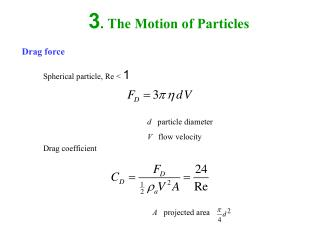Download Presentation3. The Motion of Particles

Loading in 2 Seconds...

# 3. The Motion of Particles - PowerPoint PPT Presentation

3. The Motion of Particles. Drag force. Spherical particle, Re < 1. d particle diameter V flow velocity. Drag coefficient. A projected area. Case 1: With slip. is Cunningham correction factor. For d > 0.1 m m. For d > 0.01 m m. Case 2: High Re, Re > 1.I am the owner, or an agent authorized to act on behalf of the owner, of the copyrighted work described.
Download Presentation## 3. The Motion of Particles

An Image/Link below is provided (as is) to download presentation

Download Policy: Content on the Website is provided to you AS IS for your information and personal use and may not be sold / licensed / shared on other websites without getting consent from its author.While downloading, if for some reason you are not able to download a presentation, the publisher may have deleted the file from their server.

- - - - - - - - - - - - - - - - - - - - - - - - - - E N D - - - - - - - - - - - - - - - - - - - - - - - - - -
Presentation Transcript
1. 3. The Motion of Particles Drag force Spherical particle, Re < 1 d particle diameter V flow velocity Drag coefficient A projected area

2. Case 1: With slip is Cunningham correction factor For d > 0.1 mm For d > 0.01 mm

3. Case 2: High Re, Re > 1

4. Case 3: Nonspherical particle is shape factor is equivalent volume diameter Shape/type spherical fiber (L/d = 4) quartz dust fused alumina talcum (platelet) 1 1.32 (axis perpendicular to flow) 1.07 (axis parallel to flow) 1.36 1.04-1.49 2.04

5. Motion under gravity

6. Equation of motion Particle relaxation time or time constant Terminal settling velocity

7. Mechanical mobility Terminal settling velocity with slip, shape factor

8. Motion under electrical forces q particle charge n number of charge e electron charge = 1.6x10-19 C E electric field

9. In equilibrium Terminal electrical velocity Electrical mobility

10. Relation between VTE and E for two particle sizes

11. Motion under thermal gradients Thermophoretic force -> Temperature gradient Thermophoretic velocity

12. Motion under no external force Equation of motion Velocity Traveling distance

13. Stopping distance, t >>t

14. Similarity in particle motion 1. Reynolds number (Re) must be equal 2. Stokes number (Stk) must be equal With slip

15. Particle motion for several values of Stokes number

16. 3. When gravity is important, gravitational parameter (G) must be equal To determine if inertia or gravity is more important, use Froude number (Fr)

17. Aerodynamic diameter Aerodynamic diameter (da) is the diameter of a spherical particle of density r0 = 1 g/cm3 which has the same terminal settling velocity in air as the particle of interest. Stokes diameter (ds) is the diameter of a spherical particle that has the same density and terminal settling velocity in air as the particle of interest. is the bulk density

18. Comparison of equivalent volume diameter, Stokes diameter, and aerodynamic diameter.

19. Inertial impaction Stokes number is the jet diameter

20. Collection efficiency characteristics of an impactor

21. Diffusion (Brownian motion) Random motion of an aerosol particle in still air Fick’s first law is the particle flux (# particles per unit area per unit time) is the diffusion coefficient is the number of particles is the direction of motion Stokes-Einstein derivation

22. RMS and average velocity

23. Deposition by diffusion Aerosol particle collides and sticks to the surface Fick’s second law Boundary and initial conditions

24. Solution Concentration profile for a stagnant aerosol of 0.05-mm particles near a wall

25. General form of the concentration profile near a wall

26. Cumulative number of particle deposited per unit area during time t Deposition velocity: velocity that particles move to a surface and is analogous to the terminal settling velocity due to gravity.

27. Cumulative deposition of particles on a horizontal surface during 100 sec.

28. Diffusion of aerosol particles on the tube wall Penetration for circular tube Deposition parameter is the length of the tube is the diameter of the tube is the average velocity is the flow rate

29. Penetration for rectangular tube Peclet number: another dimensionless parameter used in diffusion motion is the characteristic length

30. Penetration of aerosol particles in a tube.

31. Fractional loss to the walls by diffusion for an aerosol flowing through a 1-m-long tube